Author: Oscar Cronquist Article last updated on January 02, 2019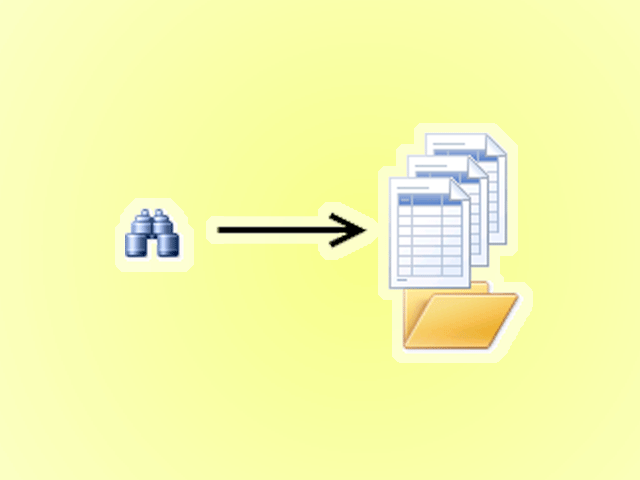Today I'll show you how to search all Excel workbooks with file extensions xls, xlsx and xlsm in a given folder for a specific string.

You can search workbooks with other file extensions as well, I will demonstrate what to change in the macro, later in this post.

The macro creates a new worksheet containing the search result. Each result contains a link to a cell where the search string was found.

You can then click the link and Excel opens the corresponding workbook and locates the cell containing the search string.

There are also macros demonstrated here that allow you to search for multiple values and return a corresponding on the same row.

The following macro asks for a folder to search in and the search string.

### Search workbooks in folder for a single string

```
Sub SearchWKBooks()
Dim WS As Worksheet
Dim myfolder As String
Dim Str As String
Dim a As Single
Dim sht As Worksheet

Set WS = Sheets.Add

With Application.FileDialog(msoFileDialogFolderPicker)
.Show
myfolder = .SelectedItems(1) & "\"
End With

Str = Application.InputBox(prompt:="Search string:", Title:="Search all workbooks in a folder", Type:=2)

If Str = "" Then Exit Sub

WS.Range("A1") = "Search string:"
WS.Range("B1") = Str
WS.Range("A2") = "Path:"
WS.Range("B2") = myfolder
WS.Range("A3") = "Workbook"
WS.Range("B3") = "Worksheet"
WS.Range("C3") = "Cell Address"
WS.Range("D3") = "Link"

a = 0

Value = Dir(myfolder)
Do Until Value = ""
If Value = "." Or Value = ".." Then
Else
If Right(Value, 3) = "xls" Or Right(Value, 4) = "xlsx" Or Right(Value, 4) = "xlsm" Then
On Error Resume Next
Workbooks.Open Filename:=myfolder & Value, Password:="zzzzzzzzzzzz"
If Err.Number > 0 Then
WS.Range("A4").Offset(a, 0).Value = Value
WS.Range("B4").Offset(a, 0).Value = "Password protected"
a = a + 1
Else
On Error GoTo 0
For Each sht In ActiveWorkbook.Worksheets
Set c = sht.Cells.Find(Str, LookIn:=xlValues, LookAt:=xlWhole, SearchOrder:=xlByRows, SearchDirection:=xlNext)
If Not c Is Nothing Then
firstAddress = c.Address
Do
WS.Range("A4").Offset(a, 0).Value = Value
WS.Range("B4").Offset(a, 0).Value = sht.Name
WS.Range("C4").Offset(a, 0).Value = c.Address
WS.Hyperlinks.Add Anchor:=WS.Range("D4").Offset(a, 0), Address:=myfolder & Value, SubAddress:= _
sht.Name & "!" & c.Address, TextToDisplay:="Link"
a = a + 1
Set c = sht.Cells.FindNext(c)
Loop While Not c Is Nothing And c.Address <> firstAddress
End If
Next sht
End If
Workbooks(Value).Close False
On Error GoTo 0
End If
End If
Value = Dir
Loop
Cells.EntireColumn.AutoFit
End Sub
```

Back to top

### Explaining the macro

The macro loops through all files in the given folder and if the file extension matches xls, xlsx or xlsm the workbook is opened.

If the workbook is password protected the macro outputs "Password protected" for that workbook.

If it successfully opens a workbook another loop is started that searches each worksheet in the workbook for the given search string.

Back to top

### Where to put the code?

1. Copy macro (CTRL + c)
2. Go to the VB Editor (Alt + F11)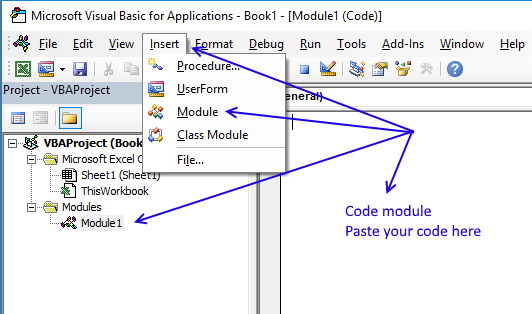3. Click "Insert" on the top menu.
4. Click "Module" to insert a code module to your workbook.
5. Paste code to the module. (CTRL + v)
6. Return to Excel.
7. Save your workbook as a macro-enabled workbook (*.xlsm file).
If you don't the macro will be gone the next time you open the same workbook.

Back to top

### Example

Example, I have 5 workbooks in folder c:\temp\ containing many random numbers. The macro asks for the path and the search string.

It then creates this sheet.Click a link and excel loads the corresponding workbook and navigates to the specific cell.

Back to top

### Search other file extensions

Change the following line to whatever file extensions you want to look for.

```If Right(Value, 3) = "xls" Or Right(Value, 4) = "xlsx" Or Right(Value, 4) = "xlsm" Then
```

Back to top

### Download Excel file

* You will also get a weekly newsletter, unsubscribe anytime!

Back to top

### Search multiple strings in all workbooks in a folder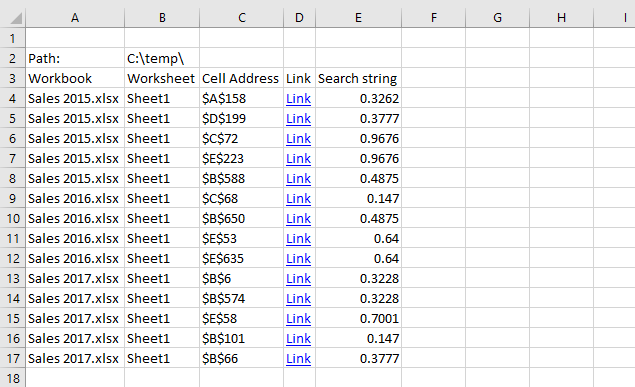The following macro allows you to search all workbooks in a folder for multiple search strings.

```Sub SearchWKBooks()
Dim WS As Worksheet
Dim myfolder As String
Dim Str As String
Dim a As Single
Dim sht As Worksheet
Dim RNG As Range

On Error Resume Next
Set RNG = Application.InputBox(Prompt:="Select a cell range containing search strings" _
, Title:="Select a range", Default:=ActiveCell.Address, Type:=8)
On Error GoTo 0

With Application.FileDialog(msoFileDialogFolderPicker)
.Show
myfolder = .SelectedItems(1) & "\"
End With

Set WS = Sheets.Add

WS.Range("A2") = "Path:"
WS.Range("B2") = myfolder
WS.Range("A3") = "Workbook"
WS.Range("B3") = "Worksheet"
WS.Range("C3") = "Cell Address"
WS.Range("D3") = "Link"
WS.Range("E3") = "Search string"

a = 0

Value = Dir(myfolder)
Do Until Value = ""
If Value = "." Or Value = ".." Then
Else
If Right(Value, 3) = "xls" Or Right(Value, 4) = "xlsx" Or Right(Value, 4) = "xlsm" Then
On Error Resume Next
Workbooks.Open Filename:=myfolder & Value, Password:="zzzzzzzzzzzz"
If Err.Number > 0 Then
WS.Range("A4").Offset(a, 0).Value = Value
WS.Range("B4").Offset(a, 0).Value = "Password protected"
a = a + 1
Else
On Error GoTo 0
For Each sht In ActiveWorkbook.Worksheets
For Each d In RNG
Set c = sht.Cells.Find(d, LookIn:=xlValues, LookAt:=xlWhole, SearchOrder:=xlByRows, SearchDirection:=xlNext)
If Not c Is Nothing Then
firstAddress = c.Address
Do
WS.Range("A4").Offset(a, 0).Value = Value
WS.Range("B4").Offset(a, 0).Value = sht.Name
WS.Range("C4").Offset(a, 0).Value = c.Address
WS.Hyperlinks.Add Anchor:=WS.Range("D4").Offset(a, 0), Address:=myfolder & Value, SubAddress:= _
sht.Name & "!" & c.Address, TextToDisplay:="Link"
WS.Range("E4").Offset(a, 0).Value = d
a = a + 1
Set c = sht.Cells.FindNext(c)
Loop While Not c Is Nothing And c.Address <> firstAddress
End If
Next d
Next sht
End If
Workbooks(Value).Close False
On Error GoTo 0
End If
End If
Value = Dir
Loop
Cells.EntireColumn.AutoFit
End Sub
```

### How to use the macro

The macro asks for a cell range containing the search strings you want to look for. The next step is which folder to look in?

A new sheet is inserted and populated with data if values are found.

### Download Excel file

* You will also get a weekly newsletter, unsubscribe anytime!

### Search multiple strings and return corresponding value(s) on the same row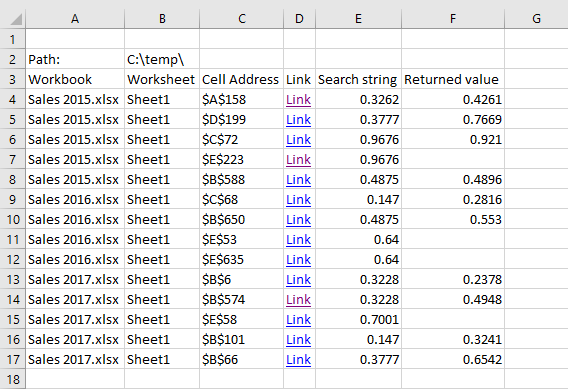The macro demonstrated above looks for multiple search strings in all Excel workbooks in a folder and also returns a value on the same row.

```Sub SearchWKBooks()
Dim WS As Worksheet
Dim myfolder As String
Dim Str As String
Dim a As Single
Dim sht As Worksheet
Dim RNG As Range

On Error Resume Next
Set RNG = Application.InputBox(prompt:="Select a cell range containing search strings" _
, Title:="Select a range", Default:=ActiveCell.Address, Type:=8)
On Error GoTo 0

Str = Application.InputBox(prompt:="Cell Offset:", Title:="Offset", Type:=2)

With Application.FileDialog(msoFileDialogFolderPicker)
.Show
myfolder = .SelectedItems(1) & "\"
End With

Set WS = Sheets.Add

WS.Range("A2") = "Path:"
WS.Range("B2") = myfolder
WS.Range("A3") = "Workbook"
WS.Range("B3") = "Worksheet"
WS.Range("C3") = "Cell Address"
WS.Range("D3") = "Link"
WS.Range("E3") = "Search string"
WS.Range("F3") = "Returned value"

a = 0

Value = Dir(myfolder)
Do Until Value = ""
If Value = "." Or Value = ".." Then
Else
If Right(Value, 3) = "xls" Or Right(Value, 4) = "xlsx" Or Right(Value, 4) = "xlsm" Then
On Error Resume Next
Workbooks.Open Filename:=myfolder & Value, Password:="zzzzzzzzzzzz"
If Err.Number > 0 Then
WS.Range("A4").Offset(a, 0).Value = Value
WS.Range("B4").Offset(a, 0).Value = "Password protected"
a = a + 1
Else
On Error GoTo 0
For Each sht In ActiveWorkbook.Worksheets
For Each d In RNG
'MsgBox d
Set c = sht.Cells.Find(d, LookIn:=xlValues, LookAt:=xlWhole, SearchOrder:=xlByRows, SearchDirection:=xlNext)
If Not c Is Nothing Then
firstAddress = c.Address
Do
WS.Range("A4").Offset(a, 0).Value = Value
WS.Range("B4").Offset(a, 0).Value = sht.Name
WS.Range("C4").Offset(a, 0).Value = c.Address
WS.Hyperlinks.Add Anchor:=WS.Range("D4").Offset(a, 0), Address:=myfolder & Value, SubAddress:= _
sht.Name & "!" & c.Address, TextToDisplay:="Link"
WS.Range("E4").Offset(a, 0).Value = d
WS.Range("F4").Offset(a, 0).Value = c.Offset(0, Str).Value
a = a + 1
Set c = sht.Cells.FindNext(c)
Loop While Not c Is Nothing And c.Address <> firstAddress
End If
Next d
Next sht
End If
Workbooks(Value).Close False
On Error GoTo 0
End If
End If
Value = Dir
Loop
Cells.EntireColumn.AutoFit
End Sub

```

### How to use

1. Start macro (Alt+F8)
2. Select the cell range containing search strings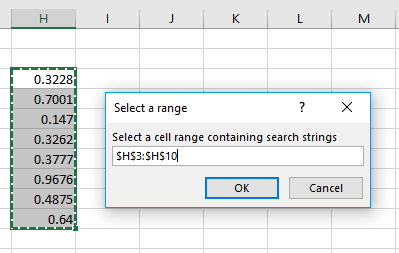3. Click OK button.
4. Select how many cells to the right (positive numbers) or to the left (negative numbers) from the found value.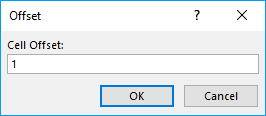Example, 1 will return the adjacent value to the right.
Example, -2 will return the value from a cell two columns to the left of the found value.
5. Select the folder you want to use.
6. Click OK

The macro will now add a worksheet to your workbook and populate it with data if values are found.Back to top

### Download Excel file

* You will also get a weekly newsletter, unsubscribe anytime!

Back to top

The following article explains how to search a folder and subfolders:

Search all workbooks in a folder and sub folders

Search all workbooks in a folder is a popular post, I am happy so many find it useful. rusl cato […]

Recommended reading:

Open Excel files in a folder [VBA]

This tutorial shows you how to list excel files in a specific folder and create adjacent checkboxes, using VBA. The […]